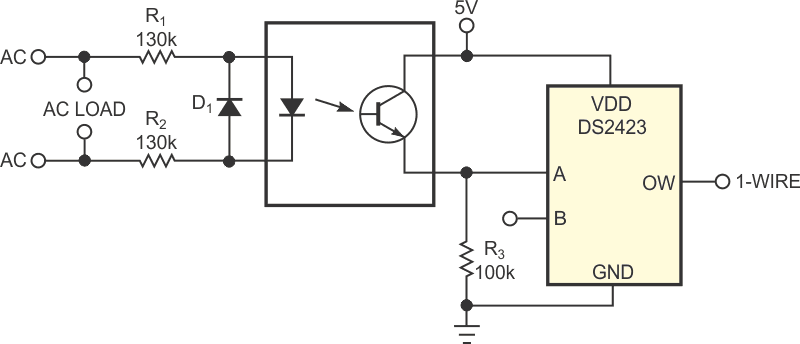# Voltage Timer Monitors Line-Connected AC Loads

## Maxim DS2423

A simple circuit monitors the elapsed time over which a line-connected ac load energizes (Figure 1). You can then access the elapsed-time count over a standard one-wire protocol. When you energize the ac load, the optoisolator provides pulses at the ac-line frequency to the input of the one-wire counter, a DS2423 IC. Thus, the counter continuously increments whenever you energize the load. Resistors R1 and R2 limit the current, and diode D1 protects the optoisolator from reverse-polarity voltages during the negative half of the line cycle.Figure 1. This circuit monitors line-connected ac loads by counting one pulse per cycle when the load is energized.

As an example, the circuit can monitor the duration of operating intervals for a 240 V-ac well pump, thereby giving an indirect measure of the amount of water the well pumps and the approximate amount of power it consumes. The one-wire master counter – a Linux-based PC, for example – reads the elapsed count once per minute. Any change in the count from one reading to the next indicates that the pump is energized and running, and you calculate the length of time in seconds by simply taking the difference in counter values divided by the line frequency – 60 Hz, in this case. The time in seconds equals the new count minus the old count divided by 60 Hz.

The circuit can monitor a water heater, a furnace, an air-conditioning unit, or any other ac-connected load. You may need to adjust the R1 and R2 values to accommodate line voltages other than 240 V ac or the characteristics of other optoisolators. You can also monitor two independent loads by attaching a second optoisolator circuit to the Counter B input of a single DS2423.

EDN